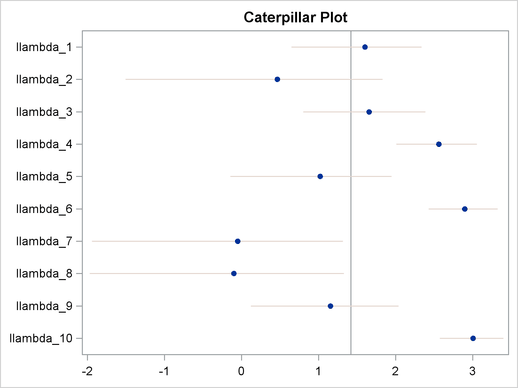Example 54.8 Nonlinear Poisson Regression Random-Effects Model

This example uses the pump failure data of Gaver and O’Muircheartaigh (1987). The number of failures and the time of operation are recorded for 10 pumps. Each of the pumps is classified into one of two groups corresponding to either continuous or intermittent operation. The following statements generate the data set:

title 'Nonlinear Poisson Regression Random-Effects Model';
data pump;
input y t group @@;
pump = _n_;
logtstd = log(t) - 2.4564900;
datalines;
5  94.320 1   1  15.720 2    5  62.880 1
14 125.760 1   3   5.240 2   19  31.440 1
1   1.048 2   1   1.048 2    4   2.096 2
22  10.480 2
;

Each row denotes data for a single pump, and the variable logtstd contains the centered operation times. Letting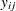denote the number of failures for theth pump in theth group, Draper (1996) considers the following hierarchical model for these data: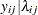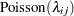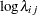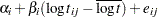The model specifies different intercepts and slopes for each group, and the random effect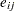is a mechanism for accounting for overdispersion. You can use noninformative priors on the parameters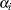,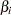, and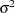, and normal prior on: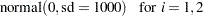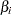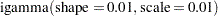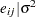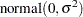The following statements fit this nonlinear hierarchical model and produce Output 54.8.1:

ods graphics on;
proc mcmc data=pump outpost=postout seed=248601 nmc=10000
plots=trace stats=none diag=none;
ods select tracepanel;
parms s2;
prior s2 ~ igamma(0.01, scale=0.01);
random alpha ~ normal(0, sd=1000) subject=group monitor=(alpha);
random beta  ~ normal(0, sd=1000) subject=group monitor=(beta);
random e     ~ normal(0, var=s2) subject=pump;
w = alpha + beta * logtstd + e;
lambda = exp(w);
model y ~ poisson(lambda);
run;

The PROC MCMC statement specifies the input data set (Pump), the output data set (Postout), a seed for the random number generator, and an MCMC sample of 10,000. The program requests that only trace plots be produced. The program also requests that posterior calculations and convergence diagnostics tests be disabled. The ODS SELECT statement displays the trace plots, and that is the primary focus.

There is only one model parameter, the variance s2 in the prior distribution for the random effect. The three following RANDOM statements specify random intercepts (alpha), random slopes (beta), and observationwise random effect e. The SUBJECT= option indicates the group indices for each random effect. The MONITOR= option selects the random-effects parameters of interest.

Next, programming statements construct the mean of the Poisson likelihood, and the MODEL statement specifies the likelihood function for each observation.

Output 54.8.1 shows trace plots for the variance parameterand the random-effects parametersand. You can see that the chains are mixing poorly.

Output 54.8.1 Trace Plots of,, andwithout Hierarchical Centering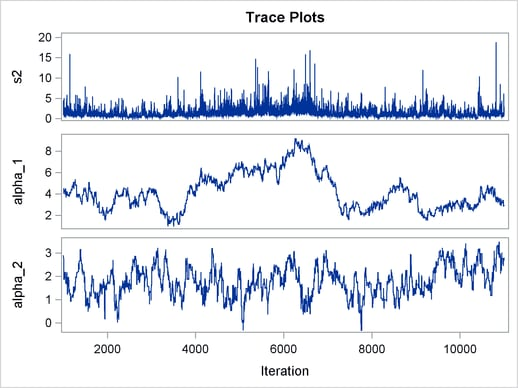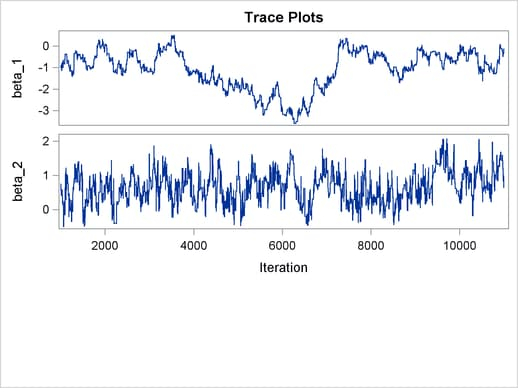To improve mixing, you can repeat the same analysis by using a hierarchical centering technique, where instead of using a normal prior centered at 0 on, you center the random effects on the group means: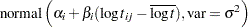Because PROC MCMC does not allow random effects to be in the prior distribution of another random effect, you cannot use the following syntax:

random alpha ~ normal(0, sd=1000) subject=group;
random beta  ~ normal(0, sd=1000) subject=group;
w = alpha + beta * logtstd;
random llambda ~ normal(w, var=s2) subject=pump;

You have to allocate arrays for the upper-level random effectsandand treat them as model parameters by declaring them in the PARMS statements. The following program illustrates how to fit a multilevel hierarchical centering random-effects model:

proc mcmc data=pump outpost=postout_c seed=248601 nmc=10000
plots=trace;
ods select tracepanel postsummaries postintervals;
array alpha;
array beta;
parms (alpha: beta:) 1 s2 1;
prior alpha: beta: ~ normal(0, sd=1000);
prior s2 ~ igamma(0.01, scale=0.01);
w = alpha[group] + beta[group] * logtstd;
random llambda ~ normal(w, var = s2) subject=pump;
lambda = exp(llambda);
model y ~ poisson(lambda);
run;

The difference between this program and the previous one is that alpha and beta are no longer declared as random effects in the RANDOM statements. Instead, you use ARRAY statements to allocate two arrays of size 2: one for alpha and one for beta. They are now treated as model parameters. You use the PARMS statement to declare the model parameters, and you use PRIOR statements to specify the prior distribuions. The symbol w is the mean of the normal distribution for the random effect llambda. Note that the data set variable group is used in alpha[group] and beta[group] as a way to select the appropriate intercept and slope for each random effect llambda.

The symbol lambda is the exponential of the corresponding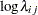(llambda), and the MODEL statement gives the response variable y a Poisson likelihood with a mean parameter lambda, in the same manner as you saw previously.

The trace plots of,, andare shown in Output 54.8.2. The mixing is significantly improved over the previous model. The posterior summary and interval statistics tables are shown in Output 54.8.1.

Output 54.8.2 Trace Plots of,, andwith Hierarchical Centering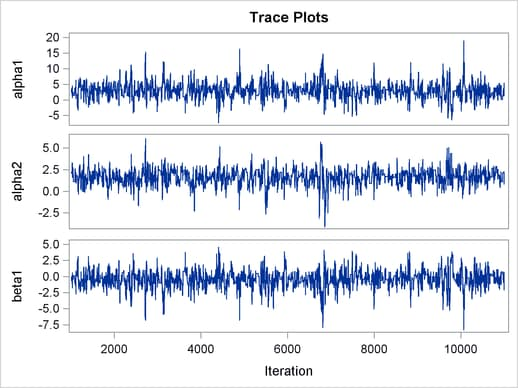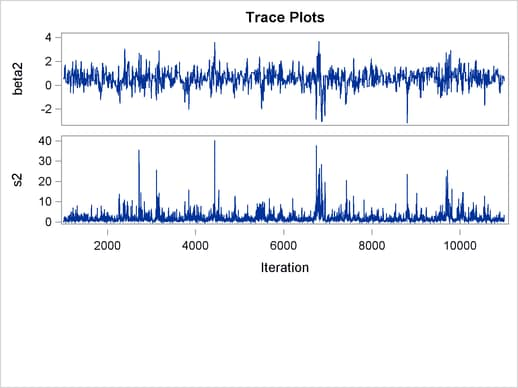Output 54.8.3 Posterior Summary Statistics
 Nonlinear Poisson Regression Random-Effects Model

The MCMC Procedure

Posterior Summaries
Parameter N Mean Standard
Deviation
Percentiles
25% 50% 75%
alpha1 10000 2.9155 2.4284 1.4939 2.9239 4.1783
alpha2 10000 1.6013 0.8997 1.0924 1.6570 2.1848
beta1 10000 -0.4282 1.3216 -1.1022 -0.4104 0.3000
beta2 10000 0.5612 0.6271 0.2086 0.5753 0.9304
s2 10000 1.7926 2.0767 0.7231 1.1842 2.0505

Posterior Intervals
Parameter Alpha Equal-Tail Interval HPD Interval
alpha1 0.050 -2.0883 8.2255 -2.1392 7.9537
alpha2 0.050 -0.2379 3.1588 -0.2082 3.1653
beta1 0.050 -3.3218 2.3148 -3.0057 2.5151
beta2 0.050 -0.6950 1.8336 -0.6555 1.8502
s2 0.050 0.3051 7.3173 0.1287 5.2457

You can easily generate a caterpillar plot (Output 54.8.4) of the random-effects parameters by calling the %CATER macro:

%CATER(data=postout_c, var=llambda_:);
ods graphics off;

Varying llambda indicates nonconstant dispersion in the Poisson model.

Output 54.8.4 Caterpillar Plots of the Random-Effects Parameters